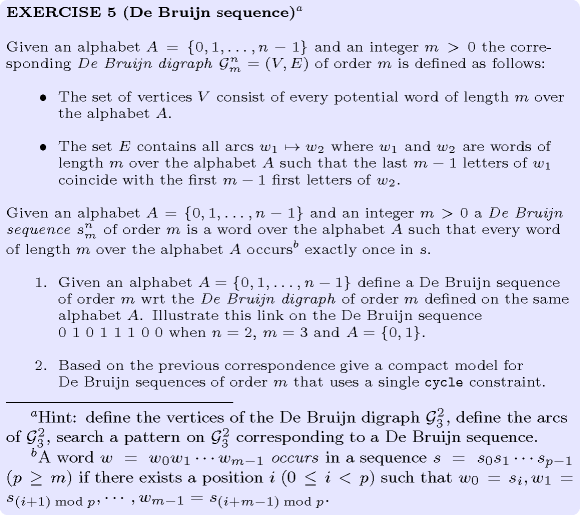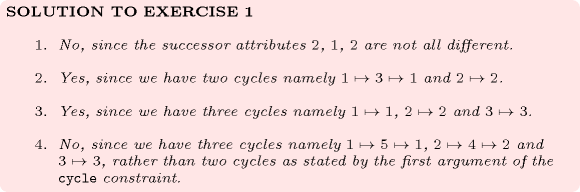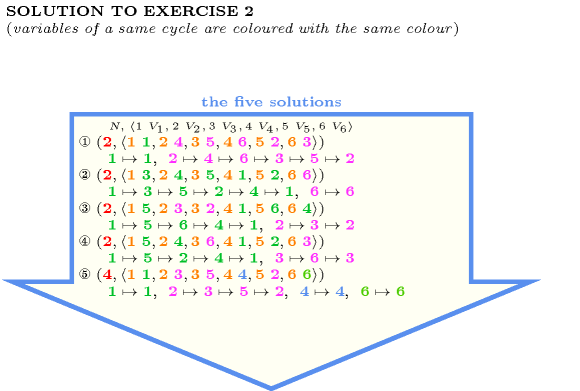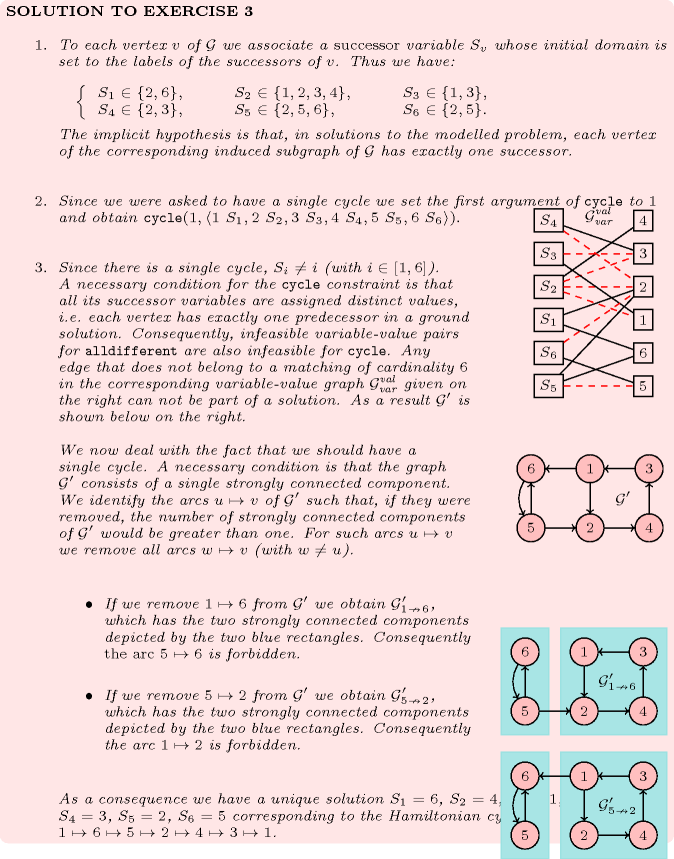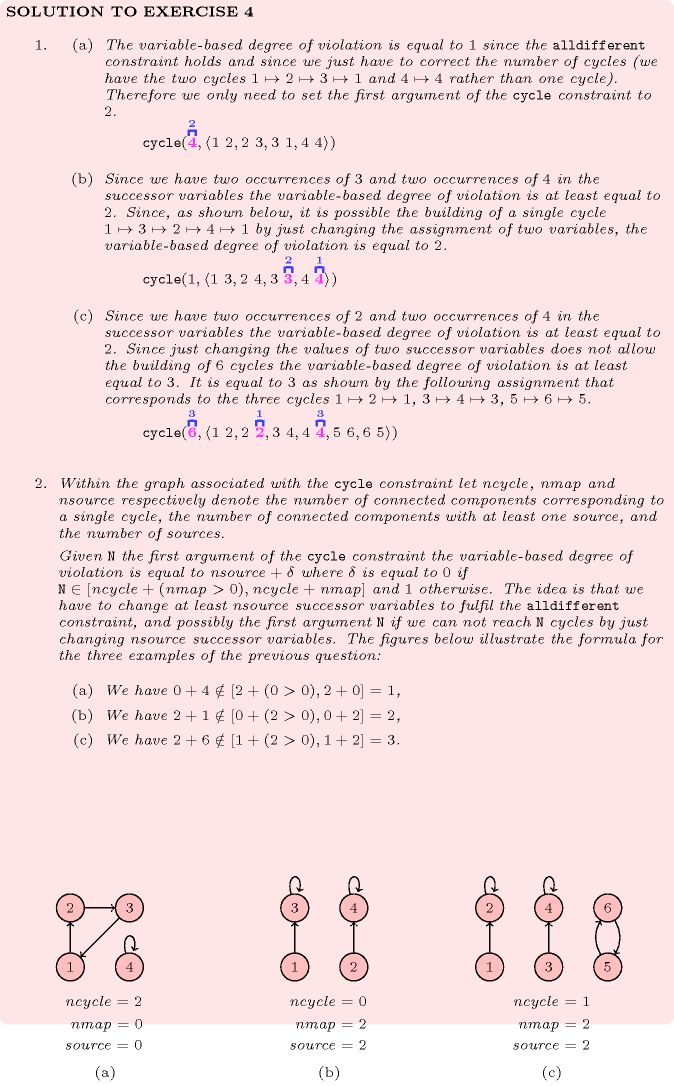## 5.103. cycle

Origin
Constraint

$\mathrm{𝚌𝚢𝚌𝚕𝚎}\left(\mathrm{𝙽𝙲𝚈𝙲𝙻𝙴},\mathrm{𝙽𝙾𝙳𝙴𝚂}\right)$

Arguments
 $\mathrm{𝙽𝙲𝚈𝙲𝙻𝙴}$ $\mathrm{𝚍𝚟𝚊𝚛}$ $\mathrm{𝙽𝙾𝙳𝙴𝚂}$ $\mathrm{𝚌𝚘𝚕𝚕𝚎𝚌𝚝𝚒𝚘𝚗}\left(\mathrm{𝚒𝚗𝚍𝚎𝚡}-\mathrm{𝚒𝚗𝚝},\mathrm{𝚜𝚞𝚌𝚌}-\mathrm{𝚍𝚟𝚊𝚛}\right)$
Restrictions
 $\mathrm{𝙽𝙲𝚈𝙲𝙻𝙴}\ge 1$ $\mathrm{𝙽𝙲𝚈𝙲𝙻𝙴}\le |\mathrm{𝙽𝙾𝙳𝙴𝚂}|$ $\mathrm{𝚛𝚎𝚚𝚞𝚒𝚛𝚎𝚍}$$\left(\mathrm{𝙽𝙾𝙳𝙴𝚂},\left[\mathrm{𝚒𝚗𝚍𝚎𝚡},\mathrm{𝚜𝚞𝚌𝚌}\right]\right)$ $\mathrm{𝙽𝙾𝙳𝙴𝚂}.\mathrm{𝚒𝚗𝚍𝚎𝚡}\ge 1$ $\mathrm{𝙽𝙾𝙳𝙴𝚂}.\mathrm{𝚒𝚗𝚍𝚎𝚡}\le |\mathrm{𝙽𝙾𝙳𝙴𝚂}|$ $\mathrm{𝚍𝚒𝚜𝚝𝚒𝚗𝚌𝚝}$$\left(\mathrm{𝙽𝙾𝙳𝙴𝚂},\mathrm{𝚒𝚗𝚍𝚎𝚡}\right)$ $\mathrm{𝙽𝙾𝙳𝙴𝚂}.\mathrm{𝚜𝚞𝚌𝚌}\ge 1$ $\mathrm{𝙽𝙾𝙳𝙴𝚂}.\mathrm{𝚜𝚞𝚌𝚌}\le |\mathrm{𝙽𝙾𝙳𝙴𝚂}|$
Purpose

Consider a digraph $G$ described by the $\mathrm{𝙽𝙾𝙳𝙴𝚂}$ collection. $\mathrm{𝙽𝙲𝚈𝙲𝙻𝙴}$ is equal to the number of circuits for covering $G$ in such a way that each vertex of $G$ belongs to a single circuit. $\mathrm{𝙽𝙲𝚈𝙲𝙻𝙴}$ can also be interpreted as the number of cycles of the permutation associated with the successor variables of the $\mathrm{𝙽𝙾𝙳𝙴𝚂}$ collection.

Example
 $\left(\begin{array}{c}2,〈\begin{array}{cc}\mathrm{𝚒𝚗𝚍𝚎𝚡}-1\hfill & \mathrm{𝚜𝚞𝚌𝚌}-2,\hfill \\ \mathrm{𝚒𝚗𝚍𝚎𝚡}-2\hfill & \mathrm{𝚜𝚞𝚌𝚌}-1,\hfill \\ \mathrm{𝚒𝚗𝚍𝚎𝚡}-3\hfill & \mathrm{𝚜𝚞𝚌𝚌}-5,\hfill \\ \mathrm{𝚒𝚗𝚍𝚎𝚡}-4\hfill & \mathrm{𝚜𝚞𝚌𝚌}-3,\hfill \\ \mathrm{𝚒𝚗𝚍𝚎𝚡}-5\hfill & \mathrm{𝚜𝚞𝚌𝚌}-4\hfill \end{array}〉\hfill \end{array}\right)$ $\left(\begin{array}{c}1,〈\begin{array}{cc}\mathrm{𝚒𝚗𝚍𝚎𝚡}-1\hfill & \mathrm{𝚜𝚞𝚌𝚌}-2,\hfill \\ \mathrm{𝚒𝚗𝚍𝚎𝚡}-2\hfill & \mathrm{𝚜𝚞𝚌𝚌}-5,\hfill \\ \mathrm{𝚒𝚗𝚍𝚎𝚡}-3\hfill & \mathrm{𝚜𝚞𝚌𝚌}-1,\hfill \\ \mathrm{𝚒𝚗𝚍𝚎𝚡}-4\hfill & \mathrm{𝚜𝚞𝚌𝚌}-3,\hfill \\ \mathrm{𝚒𝚗𝚍𝚎𝚡}-5\hfill & \mathrm{𝚜𝚞𝚌𝚌}-4\hfill \end{array}〉\hfill \end{array}\right)$ $\left(\begin{array}{c}5,〈\begin{array}{cc}\mathrm{𝚒𝚗𝚍𝚎𝚡}-1\hfill & \mathrm{𝚜𝚞𝚌𝚌}-1,\hfill \\ \mathrm{𝚒𝚗𝚍𝚎𝚡}-2\hfill & \mathrm{𝚜𝚞𝚌𝚌}-2,\hfill \\ \mathrm{𝚒𝚗𝚍𝚎𝚡}-3\hfill & \mathrm{𝚜𝚞𝚌𝚌}-3,\hfill \\ \mathrm{𝚒𝚗𝚍𝚎𝚡}-4\hfill & \mathrm{𝚜𝚞𝚌𝚌}-4,\hfill \\ \mathrm{𝚒𝚗𝚍𝚎𝚡}-5\hfill & \mathrm{𝚜𝚞𝚌𝚌}-5\hfill \end{array}〉\hfill \end{array}\right)$
• In the first example we have the following 2 ($\mathrm{𝙽𝙲𝚈𝙲𝙻𝙴}=2$) cycles: $1↦2↦1$ and $3↦5↦4↦3$. Consequently, the corresponding $\mathrm{𝚌𝚢𝚌𝚕𝚎}$ constraint holds.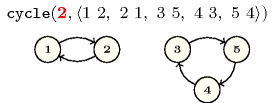• In the second example we have 1 ($\mathrm{𝙽𝙲𝚈𝙲𝙻𝙴}=1$) cycle: $1↦2↦5↦4↦3↦1$.

• In the third example we have the following 5 ($\mathrm{𝙽𝙲𝚈𝙲𝙻𝙴}=5$) cycles: $1↦1$, $2↦2$, $3↦3$, $4↦4$ and $5↦5$.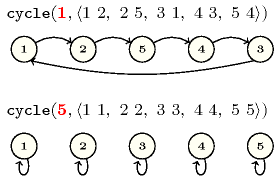All solutions

Figure 5.103.1 gives all solutions to the following non ground instance of the $\mathrm{𝚌𝚢𝚌𝚕𝚎}$ constraint: $N\in \left[1,2\right]$, ${V}_{1}\in \left[2,4\right]$, ${V}_{2}\in \left[2,3\right]$, ${V}_{3}\in \left[1,6\right]$, ${V}_{4}\in \left[2,5\right]$, ${V}_{5}\in \left[2,3\right]$, ${V}_{6}\in \left[1,6\right]$, $\mathrm{𝚌𝚢𝚌𝚕𝚎}$$\left(N,〈1{V}_{1},2{V}_{2},3{V}_{3},4{V}_{4},5{V}_{5},6{V}_{6}〉\right)$.

##### Figure 5.103.1. All solutions corresponding to the non ground example of the $\mathrm{𝚌𝚢𝚌𝚕𝚎}$ constraint of the All solutions slot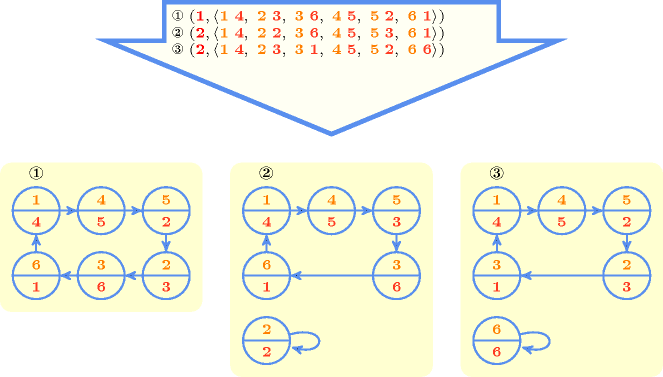Typical
 $\mathrm{𝙽𝙲𝚈𝙲𝙻𝙴}<|\mathrm{𝙽𝙾𝙳𝙴𝚂}|$ $|\mathrm{𝙽𝙾𝙳𝙴𝚂}|>2$
Symmetries
• Items of $\mathrm{𝙽𝙾𝙳𝙴𝚂}$ are permutable.

• Attributes of $\mathrm{𝙽𝙾𝙳𝙴𝚂}$ are permutable w.r.t. permutation $\left(\mathrm{𝚒𝚗𝚍𝚎𝚡},\mathrm{𝚜𝚞𝚌𝚌}\right)$ (permutation applied to all items).

Arg. properties

Functional dependency: $\mathrm{𝙽𝙲𝚈𝙲𝙻𝙴}$ determined by $\mathrm{𝙽𝙾𝙳𝙴𝚂}$.

Usage

The PhD thesis of Éric Bourreau [Bourreau99] mentions the following applications of extensions of the $\mathrm{𝚌𝚢𝚌𝚕𝚎}$ constraint:

• The balanced Euler knight problem where one tries to cover a rectangular chessboard of size $N·M$ by $C$ knights that all have to visit between $2·⌊⌊\left(N·M\right)/C⌋/2⌋$ and $2·⌈⌈\left(N·M\right)/C⌉/2⌉$ distinct locations. For some values of $N$, $M$ and $C$ there does not exist any solution to the previous problem. This is for instance the case when $N=M=C=6$. Figure 5.103.2 depicts the graph associated with the $6×6$ chessboard as well as examples of balanced solutions with respectively 1, 2, 3, 4 and 5 knights.

• Some pick-up delivery problems where a fleet of vehicles has to transport a set of orders. Each order is characterised by its initial location, its final destination and its weight. In addition one also has to take into account the capacity of the different vehicles.

##### Figure 5.103.2. Graph of potential moves of a $6×6$ chessboard, corresponding balanced knight's tours with 1 up to 5 knights, and collection of nodes passed to the $\mathrm{𝚌𝚢𝚌𝚕𝚎}$ constraint corresponding to the solution with 5 knights; note that their is no balanced knight's tour on a $6×6$ chessboard where each knight exactly performs 6 moves.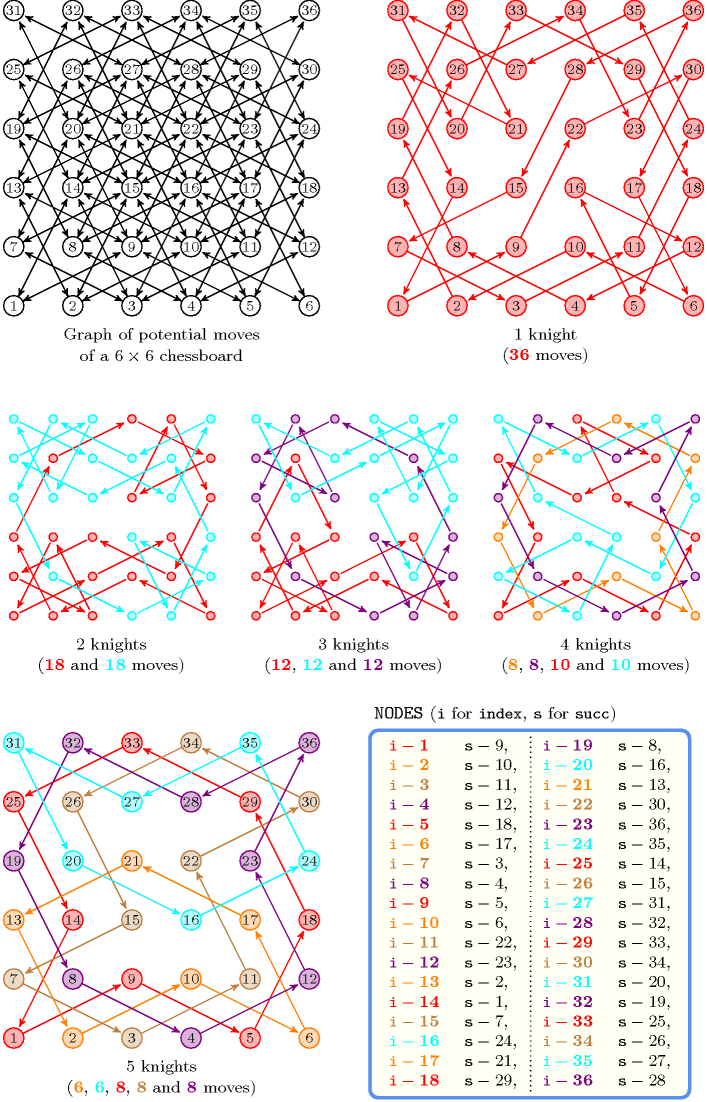Remark

In the original $\mathrm{𝚌𝚢𝚌𝚕𝚎}$ constraint of CHIP the $\mathrm{𝚒𝚗𝚍𝚎𝚡}$ attribute was not explicitly present. It was implicitly defined as the position of a variable in a list.

In an early version of the CHIP there was a constraint named $\mathrm{𝚌𝚒𝚛𝚌𝚞𝚒𝚝}$ that, from a declarative point of view, was equivalent to $\mathrm{𝚌𝚢𝚌𝚕𝚎}\left(1,\mathrm{𝙽𝙾𝙳𝙴𝚂}\right)$. In ALICE [Lauriere78] the $\mathrm{𝚌𝚒𝚛𝚌𝚞𝚒𝚝}$ constraint was also present.

Given a complete digraph of $n$ vertices as well as an unrestricted number of circuits $\mathrm{𝙽𝙲𝚈𝙲𝙻𝙴}$, the total number of solutions to the corresponding $\mathrm{𝚌𝚢𝚌𝚕𝚎}$ constraint corresponds to the sequence A000142 of the On-Line Encyclopaedia of Integer Sequences [Sloane10]. Given a complete digraph of $n$ vertices as well as a fixed number of circuits $\mathrm{𝙽𝙲𝚈𝙲𝙻𝙴}$ between 1 and $n$, the total number of solutions to the corresponding $\mathrm{𝚌𝚢𝚌𝚕𝚎}$ constraint corresponds to the so called Stirling number of first kind.

Algorithm

Since all $\mathrm{𝚜𝚞𝚌𝚌}$ variables have to take distinct values one can reuse the algorithms associated with the $\mathrm{𝚊𝚕𝚕𝚍𝚒𝚏𝚏𝚎𝚛𝚎𝚗𝚝}$ constraint. A second necessary condition is to have no more than $\overline{\mathrm{𝙽𝙲𝚈𝙲𝙻𝙴}}$ strongly connected components. Pruning for enforcing this condition, as soon as we have $\overline{\mathrm{𝙽𝙲𝚈𝙲𝙻𝙴}}$ strongly connected components, can be done by forcing all strong bridges to belong to the final solution, since otherwise we would have more than $\overline{\mathrm{𝙽𝙲𝚈𝙲𝙻𝙴}}$ strongly connected components. Since all the vertices of a circuit belong to the same strongly connected component an arc going from one strongly connected component to another strongly connected component has to be removed.

Reformulation

Let $n$ and ${s}_{1},{s}_{2},\cdots ,{s}_{n}$ respectively denotes the number of vertices (i.e., $|\mathrm{𝙽𝙾𝙳𝙴𝚂}|$) and the successor variables associated with vertices $1,2,\cdots ,n$. The $\mathrm{𝚌𝚢𝚌𝚕𝚎}$ constraint can be reformulated as a conjunction of one $\mathrm{𝚊𝚕𝚕𝚍𝚒𝚏𝚏𝚎𝚛𝚎𝚗𝚝}$ constraint, $n·\left(n-1\right)$ $\mathrm{𝚎𝚕𝚎𝚖𝚎𝚗𝚝}$ constraints, $n$ $\mathrm{𝚖𝚒𝚗𝚒𝚖𝚞𝚖}$ constraints, and one $\mathrm{𝚗𝚟𝚊𝚕𝚞𝚎}$ constraint.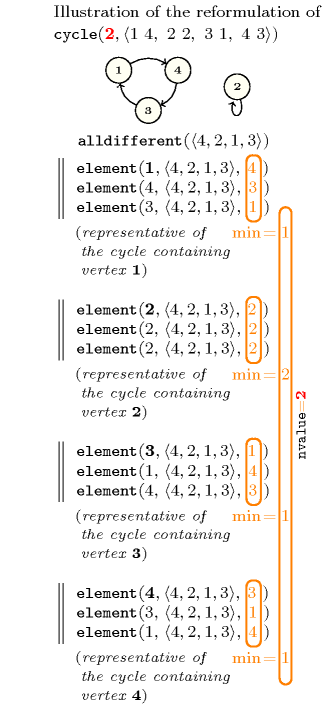Counting
 Length ($n$) 2 3 4 5 6 7 8 9 10 Solutions 2 6 24 120 720 5040 40320 362880 3628800

Number of solutions for $\mathrm{𝚌𝚢𝚌𝚕𝚎}$: domains $0..n$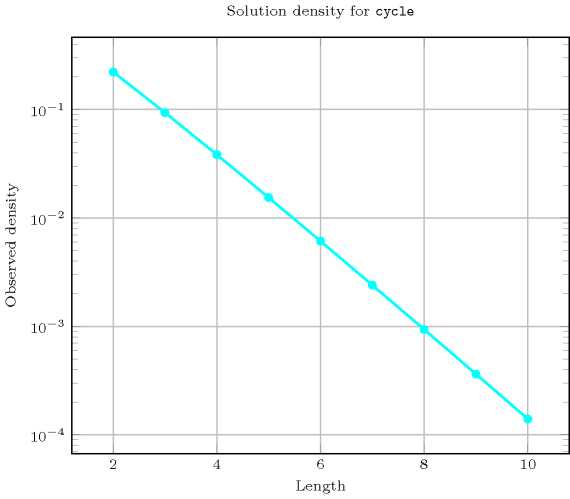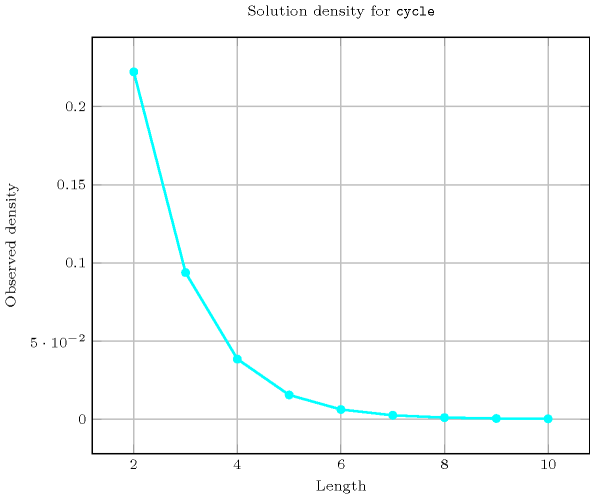Length ($n$)2345678910
Total26241207205040403203628803628800
 Parameter value

112624120720504040320362880
21311502741764130681095841026576
3-16352251624131321181241172700
4--11085735676967284723680
5---115175196022449269325
6----121322453663273
7-----1285469450
8------136870
9-------145
10--------1

Solution count for $\mathrm{𝚌𝚢𝚌𝚕𝚎}$: domains $0..n$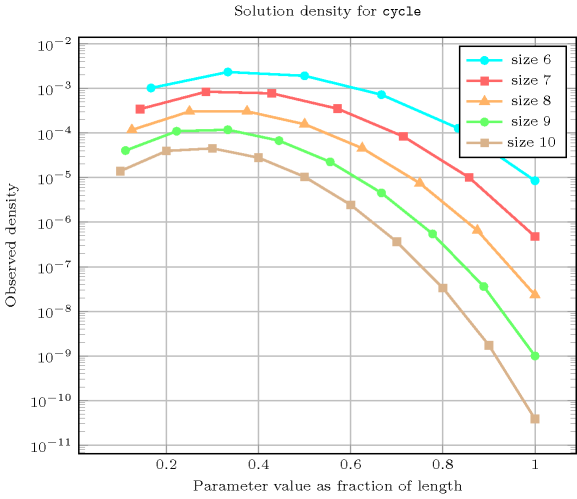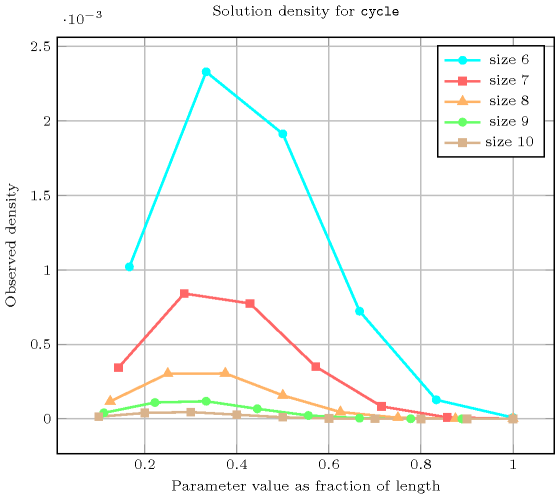related: $\mathrm{𝚋𝚊𝚕𝚊𝚗𝚌𝚎}_\mathrm{𝚌𝚢𝚌𝚕𝚎}$ (counting number of cycles versus controlling how balanced the cycles are).

specialisation: $\mathrm{𝚌𝚒𝚛𝚌𝚞𝚒𝚝}$ ($\mathrm{𝙽𝙲𝚈𝙲𝙻𝙴}$ set to 1).

Keywords
Cond. implications

$•$ $\mathrm{𝚌𝚢𝚌𝚕𝚎}\left(\mathrm{𝙽𝙲𝚈𝙲𝙻𝙴},\mathrm{𝙽𝙾𝙳𝙴𝚂}\right)$

with  $\mathrm{𝙽𝙲𝚈𝙲𝙻𝙴}=1$

implies $\mathrm{𝚋𝚊𝚕𝚊𝚗𝚌𝚎}_\mathrm{𝚌𝚢𝚌𝚕𝚎}$$\left(\mathrm{𝙱𝙰𝙻𝙰𝙽𝙲𝙴},\mathrm{𝙽𝙾𝙳𝙴𝚂}\right)$

when  $\mathrm{𝙱𝙰𝙻𝙰𝙽𝙲𝙴}=0$.

$•$ $\mathrm{𝚌𝚢𝚌𝚕𝚎}\left(\mathrm{𝙽𝙲𝚈𝙲𝙻𝙴},\mathrm{𝙽𝙾𝙳𝙴𝚂}\right)$

implies $\mathrm{𝚙𝚎𝚛𝚖𝚞𝚝𝚊𝚝𝚒𝚘𝚗}$$\left(\mathrm{𝚅𝙰𝚁𝙸𝙰𝙱𝙻𝙴𝚂}:\mathrm{𝙽𝙾𝙳𝙴𝚂}\right)$.

Arc input(s)

$\mathrm{𝙽𝙾𝙳𝙴𝚂}$

Arc generator
$\mathrm{𝐶𝐿𝐼𝑄𝑈𝐸}$$↦\mathrm{𝚌𝚘𝚕𝚕𝚎𝚌𝚝𝚒𝚘𝚗}\left(\mathrm{𝚗𝚘𝚍𝚎𝚜}\mathtt{1},\mathrm{𝚗𝚘𝚍𝚎𝚜}\mathtt{2}\right)$

Arc arity
Arc constraint(s)
$\mathrm{𝚗𝚘𝚍𝚎𝚜}\mathtt{1}.\mathrm{𝚜𝚞𝚌𝚌}=\mathrm{𝚗𝚘𝚍𝚎𝚜}\mathtt{2}.\mathrm{𝚒𝚗𝚍𝚎𝚡}$
Graph property(ies)
 $•$$\mathrm{𝐍𝐓𝐑𝐄𝐄}$$=0$ $•$$\mathrm{𝐍𝐂𝐂}$$=\mathrm{𝙽𝙲𝚈𝙲𝙻𝙴}$

Graph class
$\mathrm{𝙾𝙽𝙴}_\mathrm{𝚂𝚄𝙲𝙲}$

Graph model

From the restrictions and from the arc constraint, we deduce that we have a bijection from the successor variables to the values of interval $\left[1,|\mathrm{𝙽𝙾𝙳𝙴𝚂}|\right]$. With no explicit restrictions it would have been impossible to derive this property.

In order to express the binary constraint that links two vertices one has to make explicit the identifier of the vertices. This is why the $\mathrm{𝚌𝚢𝚌𝚕𝚎}$ constraint considers objects that have two attributes:

• One fixed attribute $\mathrm{𝚒𝚗𝚍𝚎𝚡}$ that is the identifier of the vertex,

• One variable attribute $\mathrm{𝚜𝚞𝚌𝚌}$ that is the successor of the vertex.

The graph property $\mathrm{𝐍𝐓𝐑𝐄𝐄}$ $=$ 0 is used in order to avoid having vertices that both do not belong to a circuit and have at least one successor located on a circuit. This concretely means that all vertices of the final graph should belong to a circuit.

Parts (A) and (B) of Figure 5.103.3 respectively show the initial and final graph associated with the first example of the Example slot. Since we use the $\mathrm{𝐍𝐂𝐂}$ graph property, we show the two connected components of the final graph. The constraint holds since all the vertices belong to a circuit (i.e., $\mathrm{𝐍𝐓𝐑𝐄𝐄}$ $=$ 0) and since $\mathrm{𝙽𝙲𝚈𝙲𝙻𝙴}$ $=$ $\mathrm{𝐍𝐂𝐂}$ $=$ 2.

##### Figure 5.103.3. Initial and final graph of the $\mathrm{𝚌𝚢𝚌𝚕𝚎}$ constraint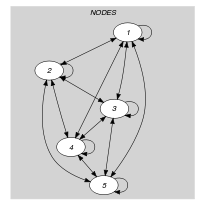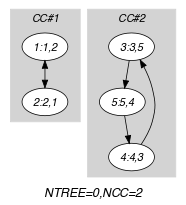(a) (b)
Quiz

$\mathrm{𝚌𝚢𝚌𝚕𝚎}$: checking whether a ground instance holds or not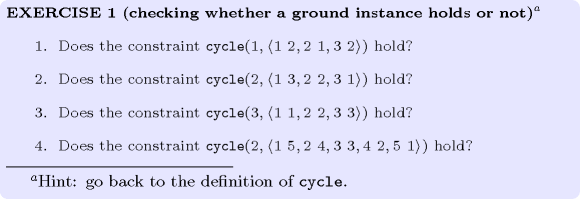$\mathrm{𝚌𝚢𝚌𝚕𝚎}$: finding all solutions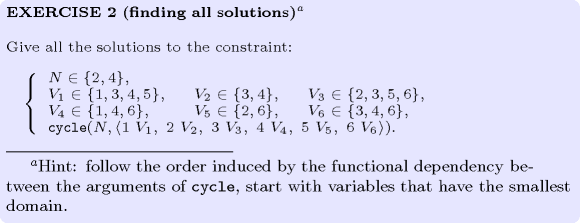$\mathrm{𝚌𝚢𝚌𝚕𝚎}$: identifying infeasible values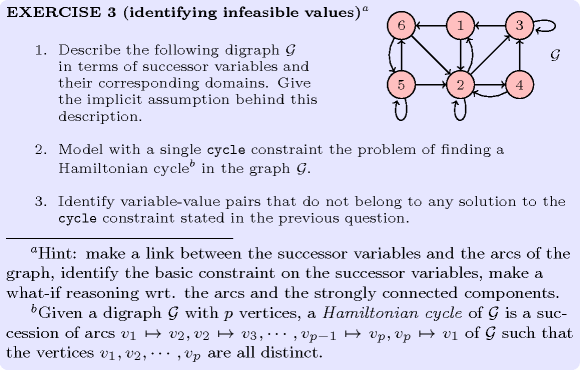$\mathrm{𝚌𝚢𝚌𝚕𝚎}$: variable-based degree of violation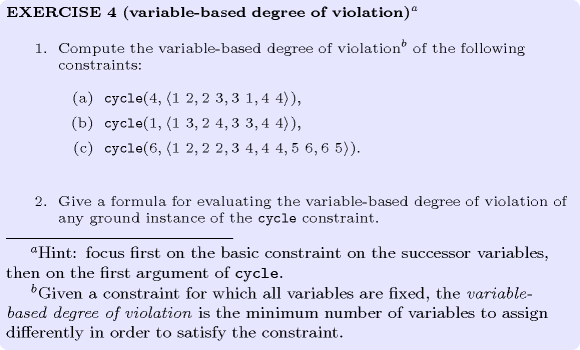$\mathrm{𝚌𝚢𝚌𝚕𝚎}$: De Bruijn sequence